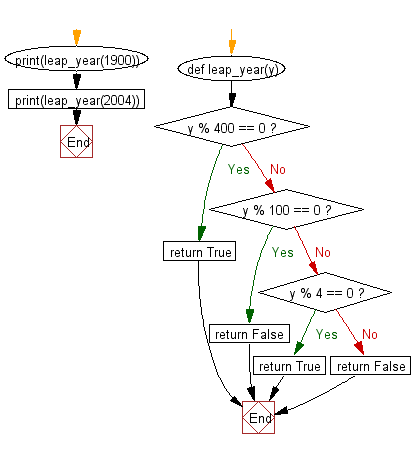﻿ Python: Determine whether a given year is a leap year - w3resource# Python: Determine whether a given year is a leap year

## Python datetime: Exercise-2 with Solution

Write a Python program to determine whether a given year is a leap year.

Sample Solution:

Python Code:

``````def leap_year(y):
if y % 400 == 0:
return True
if y % 100 == 0:
return False
if y % 4 == 0:
return True
else:
return False
print(leap_year(1900))
print(leap_year(2004))
```
```

Sample Output:

```False
True
```

Flowchart:## Visualize Python code execution:

The following tool visualize what the computer is doing step-by-step as it executes the said program:

Python Code Editor:

What is the difficulty level of this exercise?

Test your Python skills with w3resource's quiz

﻿

## Python: Tips of the Day

Free up Memory:

• Manual garbage collection can be performed on timely or event based mechanism.
```import gc
collected_objects = gc.collect()
```